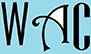## Solving triangles, equilateral triangles, hypotenuse, & triangle angles

This Webpage is for disadvantaged students in special education programs & special education with special needs and college students who need online assistance in solving trigonometry questions successfully. These are popular trig questions in solving triangles, equilateral triangles, hypotenuse, triangle angles, & what is the value of sides that they can work on or refer to in order to do their trigonometry homework or answer trigonometry problems on their trigonometry worksheets.

We provide trig online tutor for high school and college students, especially those from low income backgrounds, to help them with their trigonometry homework online.  We have provided trigonometry online questions and answers to assist students with their trigonometry practice, trigonometry study, trigonometry homework, as well as trig tests and quizzes in solving triangles, equilateral triangles, hypotenuse, triangle angles, & what is the value of sides .

These trigonometry online questions below also have answer for the trigonometry online when you click on each link.  Here are a list of the most popular and most common trig online equations that can be found in many trigonometry homework and trigonometry exams on solving triangles, equilateral triangles, hypotenuse, triangle angles, & what is the value of sides.  The answers for the trigonometry online problems are explained in steps and the trigonometry online functions are easily understood.